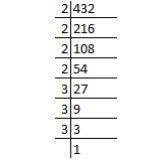# NCERT Exemplar Solutions Class 7 Mathematics Solutions for Exponents and Powers - Exercise in Chapter 11 - Exponents and Powers

432 = 24 × 3 ________.

432 = 24 × 33

Now, we have to find out the factors of 432.Therefore, factors of 432 are = 2 × 2 × 2 × 2 × 3 × 3 × 3

432 = 24 × 3 3

Related Questions
Exercises

Lido

Courses

Teachers

Book a Demo with us

Syllabus

Maths
CBSE
Maths
ICSE
Science
CBSE

Science
ICSE
English
CBSE
English
ICSE
Coding

Terms & Policies

Selina Question Bank

Maths
Physics
Biology

Allied Question Bank

Chemistry
Connect with us on social media!